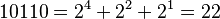# Binary number facts for kids

Kids Encyclopedia Facts

The binary numeral system is a way to write numbers using only two digits: 0 and 1. These are used in computers as a series of "off" and "on" switches. 0 is "off" and 1 is "on." In binary, each digit's place value is twice as much as that of the next digit to the right (since each digit holds two values). In decimal — the system that humans normally use — each digit holds ten values, and the place value increases by a power of ten (ones, tens, hundreds, etc.). The place value of the rightmost digit, in either case, is 1.

Here is an example of a binary number and how it reads in Arabic numbers: 10110011

• The place value of the last 1 (rightmost position) is 1.
• The place value of the 1 before that is 2.
• The place value of the 0 before that is 4.
• The place value of the 0 before that is 8.
• The place value of the 1 before that is 16.
• The place value of the 1 before that is 32.
• The place value of the 0 before that is 64.
• The place value of the 1 before that is 128.

Adding together all the place values that have 1s, it would be 1+2+16+32+128 = 179.

 0 0000 0+0+0+0 1 0001 0+0+0+1 2 0010 0+0+2+0 3 0011 0+0+2+1 4 00100 0+0+4+0+0 5 00101 0+0+4+0+1 6 00110 0+0+4+2+0 7 00111 0+0+4+2+1 8 01000 0+8+0+0+0 9 01001 0+8+0+0+1 10 01010 0+8+0+2+0 11 01011 0+8+0+2+1 12 01100 0+8+4+0+0 13 01101 0+8+4+0+1 14 01110 0+8+4+2+0 15 01111 0+8+4+2+1 16 10000 16+0+0+0+0 17 10001 16+0+0+0+1 18 10010 16+0+0+2+0 19 10011 16+0+0+2+1 20 10100 16+0+4+0+0 21 10101 16+0+4+0+1 22 10110 16+0+4+2+0 23 10111 16+0+4+2+1 24 11000 16+8+0+0+0 25 11001 16+8+0+0+1 26 11010 16+8+0+2+0 27 11011 16+8+0+2+1 28 11100 16+8+4+0+0 29 11101 16+8+4+0+1 30 11110 16+8+4+2+0 31 11111 16+8+4+2+1

For convenience, binary digits (bits, for short) are usually grouped into two groups of 4 bits. This is 8 bits, or a byte, and is written using the hexadecimal numeral system.

## Binary arithmetic

Arithmetic is a way of adding together two or more binary numbers. There are four rules in binary arithmetic. They are:

0 + 0 = 0
1 + 0 = 1
1 + 1 = 10 (2)
1 + 1 + 1 = 11 (3)

This is because in binary there are only two digits: 0 and 1. Because of this, the numbers two and three have to be represented in some other way. This is how the binary value for three is calculated:

Column Decimal Value Binary
1 2 1
2 1 1

This shows that the binary value would be 11.

## History

Binary is a numbering system that is a series of 1s and 0s meaning (to the computers) on and off. It is base 2 and our number system (decimal) is base 10, where 10 numerals are used rather than 2 (see the first chart).

Binary was invented by many people, but the modern binary number system is credited to Gottfried Leibniz in 1679, a German mathematician. Binary has been used in nearly everything electronic; from calculators to supercomputers. Machine code is binary digits.

In 1817, John Leslie (a Scottish mathematician) suggested that primitive societies may have used objects like pebbles before they had words to describe the total number of a group of objects. It is possible that they could have divided the objects into piles of equal numbers with a remainder of either 0 or 1. They could have continued to divide the piles into equal parts with a remainder of either 0 or 1.

## Translation

You can translate binary to normal numbers using$10110 = 2^4 + 2^2 + 2^1 =22$

## Images for kidsIn Spanish: Sistema binario para niños